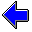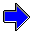Java Security Settings:
This web page employs Java, which requires specific security settings for correct operation.
If the applets on this page do not run correctly, consult the Virtual Chemistry Experiments FAQ
or the Physlet Physics web site for establishing the correct security settings.

Calorimetry

Heat of Solution of Sulfuric Acid

Concepts

The objective of this experiment is to determine the heat of solution of sulfuric acid.

H2SO4 (l)   →   H+ (aq) + HSO4- (aq)

The procedure for this experiment is similar to that for determining the heat of solution of ammonium nitrate. A small glass bulb containing pure sulfuric acid is placed in a calorimeter containing water. When the bulb is broken, the sulfuric acid dissolves in the water, and the heat from the reaction is released to the water and the calorimeter.

This experiment adds a degree of realism to the experimental procedure. To this point, it has been assumed that the calorimeter is perfectly insulated and no heat enters or leaves the system from the surroundings. In practice, the insulation is not perfect and there is some heat loss or gain.

If the system is at room temperature initially, the initial temperature of the system will be stable. The final temperature, however, will not be at room temperature. If the reaction is exothermic, the final temperature will be above room temperature, and heat will slowly be lost from the calorimeter to the surroundings. Instead of being constant, the final temperature will slowly drift downward. The converse is true for an endothermic reaction.

To compensate for this heat loss/gain, the temperature is measured as a function of time. Prior to the initiation of the chemical reaction, the temperature vs time plot can be used to establish the initial baseline. When the reaction is complete, the temperature vs time plot can be used to establish the final baseline. These baselines are typically linear, especially if the calorimeter is well-insulated, which makes the heat loss/gain relatively small. The baselines represent the temperature changes attributable to heat exchange from the surroundings.

To determine Ti and Tf, imagine that the reaction took place instantaneously and the heat from the reaction is instantly distributed evenly throughout the system. In this hypothetical case, the temperature would instantaneously jump from Ti to Tf at the time the reaction was initiated. These temperatures cannot be measured directly, but they can be inferred by extrapolating the baselines to obtain the temperatures on the initial and final baselines at the time the reaction was initiated. (Strictly speaking, initial and final temperature reading should be taken at the "effective" initial time, which is not exactly the time when the reaction was initiated. For the purposes of this virtual experiment, the time when the experiment was initiated will be used.)

Experiment

Objective:

• Determine the heat of solution of sulfuric acid.

Approach:

• Dissolve sulfuric acid in water in a calorimeter.
• Monitor the variation of the temperature with time.
• Determine baselines for the times before the reaction started and after the reaction finished.
• Extrapolate the baselines to the reaction time to obtain the initial and final temperatures.
• Calculate the heat of solution of sulfuric acid.

Enter values for the masses of water and sulfuric acid (FW 98.08) and start the experiment. The temperature is sampled at 0.5 sec intervals and automatically plotted on the graph. The temperature vs time data is listed in a separate window.

The heat capacity of the calorimeter is 288. J oC-1

The reaction is initiated at t = 5.00 sec, which is marked on the graph by a vertical black line.

Numerical values of the experimental data are shown in the columns at the right. Under the "Initial Baseline" column, select the points you wish to use to establish the baseline prior to initiation of the reaction. By holding down the control or shift key, it is possible to select multiple points on this list. After you have selected the points, click the "Plot Initial Baseline" button in the main browser window. The initial baseline will be displayed on the graph, and the slope and intercept will be listed. In general, the initial baseline is well-defined and linear, and you should use all of the points prior to the initiation of the reaction to construct the initial baseline.

Carefully examine the points near the end of the experiment at long times after the reaction is finished and the system is slowly cooling. Select a number of points from the linear region of the data to determine the final baseline. Click the "Plot Final Baseline" button in the main browser window to plot the final baseline.

The purpose of the baselines is to determine the initial and final temperature that would have been observed had the reaction occurred instanteously and had the heat from the reaction been distributed uniformly throughout the system instantaneously. (This is obviously a hypothetical situation.) Use the mouse to read the temperatures at t = 5.00 sec (the reaction starting time) that lie on the initial (Ti) and final (Ti) baselines. When the cursor is over the graph, press the left mouse button to obtain a crosshair cursor and to display the time and temperature at the position of the cursor. Carefully position the mouse on the desired point. Alternatively, one can use the slope and intercept for each baseline to calculate the temperature at 5.00 sec.

Use the initial and final temperatures to calculate the heat of solution of sulfuric acid.

Initial Baseline Final Baseline
Mass of Water: g

Mass of H2SO4: g

slope:     intercept:

slope:     intercept:Heat of Neutralization                     Heat of Combustion of MethaneCalorimetry Home PageVirtual Chemistry Home Page

HeatOfSolutionOfSulfuricAcid.html version 2.1